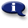# .

## Syllabus Information

Spring 2022
Dec 03,2023Use this page to maintain syllabus information, learning objectives, required materials, and technical requirements for the course.

Syllabus Information
MTH 065C - Elementary Algebra: Part C
Associated Term: Spring 2022
Learning Objectives: Upon completion of this course, the student will: 1- Maintain, use, and expand skills and concepts learned in previous mathematics courses. 2- Perform addition, subtraction, multiplication, and division of rational numbers. 3- Use and apply the concepts and language of algebraic expressions. 4- Solve linear equations and inequalities. 5- Interpret information represented numerically and graphically, and recognize the linear relationships represented verbally, numerically, graphically, and algebraically. 6- Factor polynomials. 7- Factor polynomials by removing a common monomial factor. 8- Factor trinomials. Factor special products. 9- Recognize and use quadratic equations. 10- Sketch the graph of a quadratic equation in two variables and identify the intercepts and the vertex graphically. 11- Solve a quadratic equation by factoring or by the square root property. 12- Recognize values of a variable that make an algebraic fraction undefined, and reduce algebraic fractions to lowest terms. 13- Make appropriate and efficient use of a scientific calculator. (Note: Students will be expected to demonstrate achievement of some objectives without the use of a calculator.)
Required Materials:
Technical Requirements: